# Universal enveloping algebra

(diff) ← Older revision | Latest revision (diff) | Newer revision → (diff)

of a Lie algebraover a commutative ringwith a unit element

The associative-algebra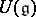with a unit element, together with a mappingfor which the following properties hold: 1)is a homomorphism of Lie algebras, i.e.is-linear and,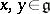; 2) for every associative-algebrawith a unit element and every-algebra mapping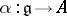such that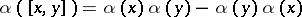,, there exists a unique homomorphism of associative algebras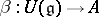, mapping the unit to the unit, such that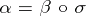. The universal enveloping algebra is unique up to an isomorphism and always exists: If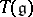is the tensor algebra of the-module,is the two-sided ideal generated by all elements of the form,, and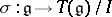is the canonical mapping, then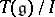is the universal enveloping algebra of.

Ifis Noetherian and the modulehas finite order, then the algebrais left and right Noetherian. Ifis a free module over an integral domain, thenhas no zero divisors. For any finite-dimensional Lie algebraover a fieldthe algebrasatisfies the Ore condition (cf. Imbedding of semi-groups) and so has a skew-field of fractions.

Ifis any-module, then every homomorphism of Lie algebrasextends to a homomorphism of associative algebras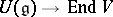. This establishes an isomorphism of the category of-modules and the category of left-modules, whose existence forms the basis for the application of universal enveloping algebras in the theory of representations of Lie algebras (cf. , ).

The universal enveloping algebra of the direct product of Lie algebras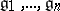is the tensor product of the algebras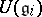. Ifis a subalgebra of, whereandare free-modules, then the canonical homomorphism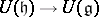is an imbedding. If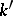is an extension of the field, then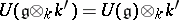. A universal enveloping algebra has a canonical filtration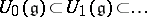, where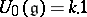and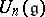for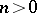is the-submodule ofgenerated by the products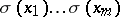,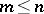,for all. The graded algebra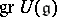associated to this filtration is commutative and is generated by the image under the natural homomorphism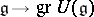; this mapping defines a homomorphismof the symmetric algebra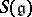of the-moduleonto. By the Poincaré–Birkhoff–Witt theorem,is an algebra isomorphism ifis a free-module. The following is an equivalent formulation: Ifis a basis of the-module, whereis a totally ordered set, then the family of monomials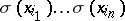,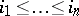,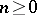, forms a basis of the-module(in particular,is injective).

Let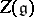be the centre of. Then for any finite-dimensional Lie algebraover a field of characteristic zero,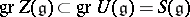consists of the subalgebra of-invariant elements in. Ifis semi-simple, thenis the algebra of polynomials invariables.

One of the important directions of research in universal enveloping algebra is the study of primitive ideals (cf. Primitive ideal; ).

How to Cite This Entry:
Universal enveloping algebra. Encyclopedia of Mathematics. URL: http://encyclopediaofmath.org/index.php?title=Universal_enveloping_algebra&oldid=18977
This article was adapted from an original article by V.L. Popov (originator), which appeared in Encyclopedia of Mathematics - ISBN 1402006098. See original article